You can show trend lines in a visualization to highlight trends in your data. You can publish a view that contains trend lines, and you add trend lines to a view as you edit it on the web.

When you add trend lines to a view, you can specify how you want them to look and behave.

For a 5-minute walkthrough, see the Trend Lines(Link opens in a new window) free training video. Use your tableau.com account to sign in. To view more training and introductory videos, go to Free Training Videos(Link opens in a new window) on the Tableau website.

## Add trend lines to a view

To add a trend line to a visualization:

1. Select the Analytics pane.

2. From the Analytics pane, drag Trend Line into the view, and then drop it on the Linear, Logarithmic, Exponential, Polynomial, or Power model types.

For more information on each of these model types, see Trend Line Model Types .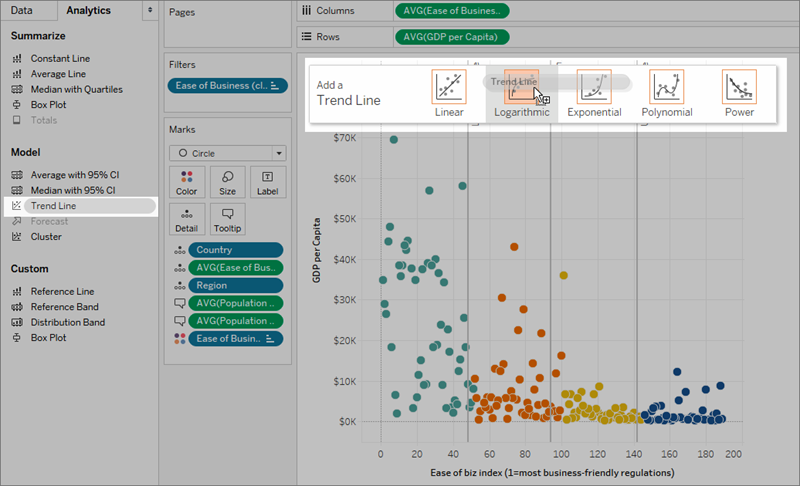To add trend lines to a view, both axes must contain a field that can be interpreted as a number. For example, you cannot add a trend line to a view that has the Product Category dimension, which contains strings, on the Columns shelf and the Profit measure on the Rows shelf. However, you can add a trend line to a view of sales over time because both sales and time can be interpreted as numeric values.

For multidimensional data sources, the date hierarchies actually contain strings rather than numbers. Therefore, trend lines are not allowed. Additionally, the ‘m/d/yy’ and ‘mmmm yyyy’ date formats on all data sources do not allow trend lines.

If you have trend lines turned on and you modify the view in a way where trend lines are not allowed, the trend lines do not show. When you change the view back to a state that allows trend lines, they reappear.

Tableau automatically stacks bar marks in many cases. However, trend lines cannot be turned on for stacked bars. You can turn off stacked marks by clearing the Analysis > Stack Marks option.

## Edit a trend line

Once you add a trend line to the visualization, you can edit it to fit your analysis.

To edit a trend line:

In Tableau Desktop: Right-click a trend line in the visualization, and select Edit Trend Lines.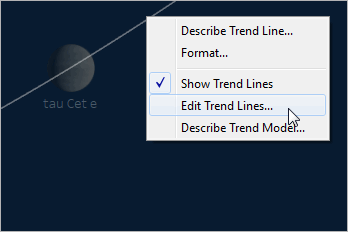In web editing mode:

1. In the visualization, click the trend line, and then hover your cursor over it.
2. In the tooltip that appears, select Edit to open the Trend Line Options dialog box.

Note: To edit a trend line in Tableau Cloud or Tableau Server, you must have web editing permissions.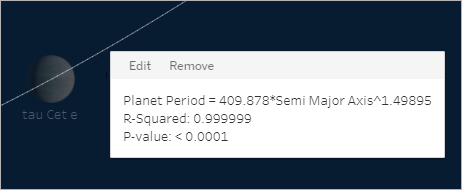You can configure the following options in the Trend Line Options dialog box:

• Select a model type. For more information, see Trend Line Model Types .

• Select which fields to use a factors in the trend line model. For more information, see Choose which fields to use as factors in the trend line model.

• Decide whether to exclude color, using the Allow a trend line per color option. When you have color encodings in your view, you can use this option to add a single trend line that models all of the data, ignoring the color encoding.
• Decide whether to Show Confidence Bands. Tableau confidence bands show upper and lower 95% confidence lines by default when you add trend lines. Confidence lines are not supported for Exponential models.

• Select whether to Force the y-intercept to zero. This option is useful when you know that you want your trend line to begin at zero. This option is available only when both the Rows shelf and the Columns shelf contain a continuous field, as with a scatterplot.

• Decide whether to show recalculated lines when you select or highlight data in the visualization.

### Choose which fields to use as factors in the trend line model

For trend models that are considering multiple fields, you can eliminate specific fields as factors in the trend line model.

Often you will want to remove factors because you want the trend line model to be based on the entire row in the table rather than broken up by the members or values of a field. Consider the following example. The view below shows the monthly sales for various products categories, broken out by region.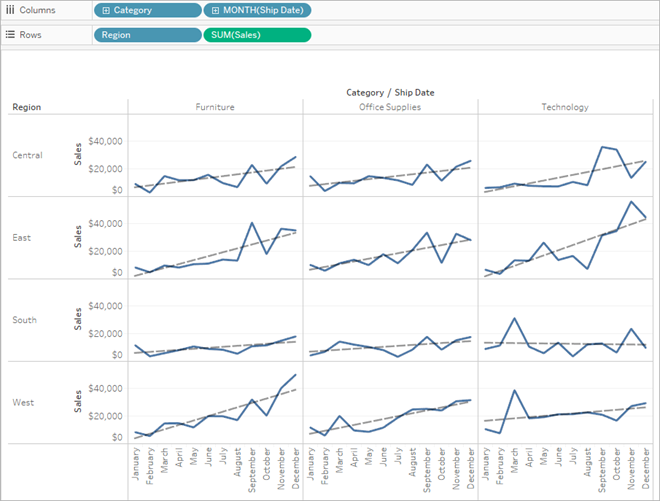You can see that a separate model is created for each region.

Now remove Region as a factor in the model by deselecting it in the Trend Lines Options dialog box.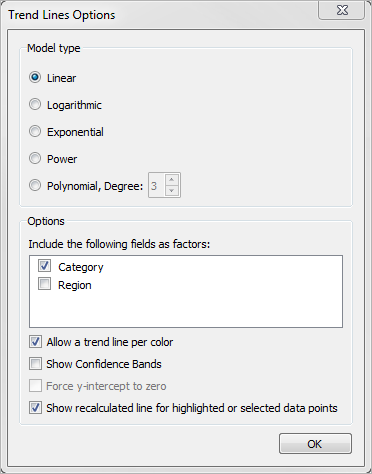You can see that the trend line model within a category is now the same across all regions. This allows you to compare actual sales against a trend line that is the same for all regions.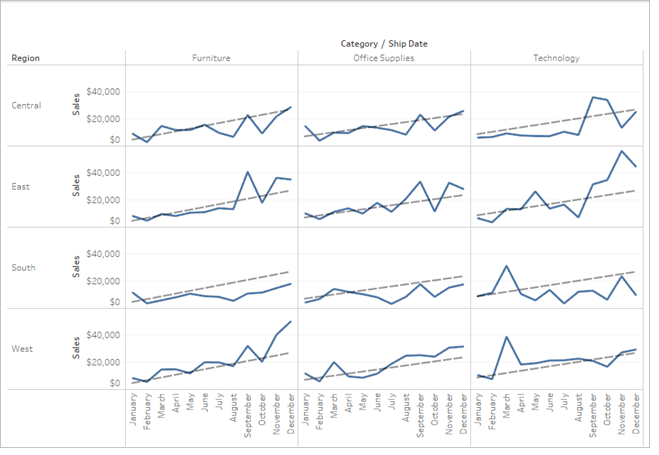## Remove Trend Lines

To remove a trend line from a visualization, drag it off of the visualization area. You can also click a trend line and select Remove.

To remove all trend lines from the view, select Analysis >Trend Lines >Show Trend Lines.

Note: In Tableau Desktop, trend line options are retained so that if you choose Show Trend Lines again from the Analysis menu, the options are as you last set them. However, if you close the workbook with trend lines turned off, trend line options revert to defaults.

## See a description of a trend line or trend line model

After you add trend lines, you can display statistics on the trend line. For example, you can see the formula as well as r-squared and p values. For more information on the model types and terms used in the descriptions, see the Trend Line Model Terms and Trend Line Model Types sections.

To see a description of a trend line:

• Hover over any part of a trend line to see its description.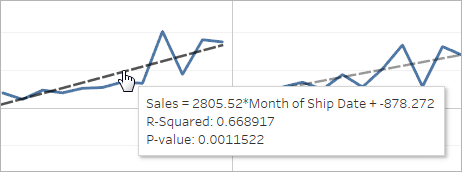Tableau Desktop only

• Right-click the trend line in the visualization , and then select Describe Trend Line.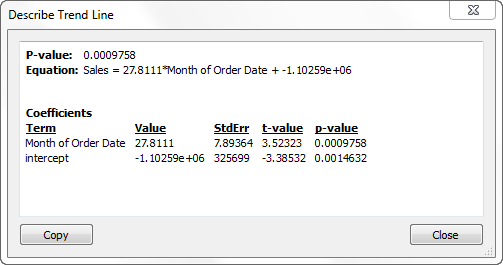To view a full description of the model being used in the current view:

• Right-click a trend line in the visualization, and then select Describe Trend Model.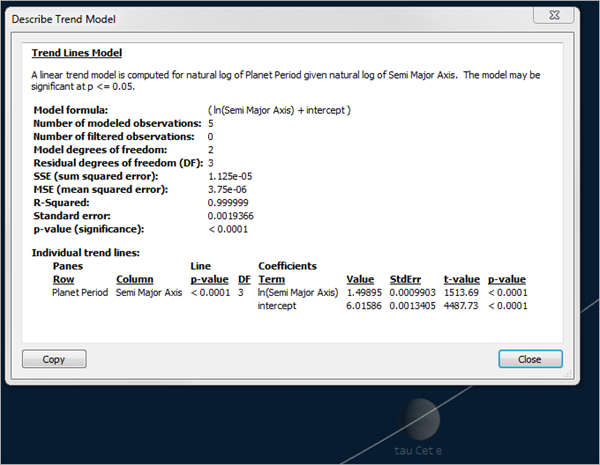## Trend Line Model Types

These model types are available for trend lines: Linear, Logarithmic, Exponential, Power, and Polynomial.

In the following formulas, X represents the explanatory variable, and Y the response variable.

### Linear

With the linear model type the formula is:

`Y = b0 + b1 * X`

where `b1` is the slope and `b0` is the intercept of the line.

### Logarithmic

With the logarithmic model type, the formula is:

`Y = b0 + b1 * ln(X)`

Because a logarithm is not defined for number less than zero, any marks for which the explanatory variable is negative are filtered before estimation of the model. Avoid using a model that discards some data unless you know that the data being filtered out is invalid. The trend line description reports how many marks were filtered before model estimation.

### Exponential

With the exponential model type, the formula is:

`Y = exp(b0)* exp(b1 * X)`

With an exponential model, the response variable is transformed by the natural log before estimation of the model so the marks plotted in your view are found by plugging in various explanatory values to find values of `ln(Y)`.

`ln(Y) = b0 + b1 * X`

These values are then exponentiated to plot the trend line. What you see is the exponential model in the following form:

`Y = b2*exp(b1 * X)`

Where `b2` is the value of `exp(b0)`. Because a logarithm is not defined for numbers less than zero, any marks for which the response variable is negative are filtered before model estimation.

### Power

With the power model type, the formula is:

`Y = b0 * X^b1`

With a power model, both variables are transformed by the natural log before estimation of the model resulting in this formula:

`ln(Y) = ln(b0) + b1 * ln(X)`

These values are then exponentiated to plot the trend line.

Because a logarithm is not defined for numbers less than zero, any marks for which the response variable or explanatory variable is negative are filtered before model estimation.

### Polynomial

With the polynomial model type, the response variable is transformed into a polynomial series of the specified degree. The formula is:

`Y = b0 + b1 * X + b2 * X^2 + …`

With a polynomial model type, you must also select a Degree between 2 and 8. The higher polynomial degrees exaggerate the differences between the values of your data. If your data increases very rapidly, the lower order terms may have almost no variation compared to the higher order terms, rendering the model impossible to estimate accurately. Also, more complicated higher order polynomial models require more data to estimate. Check the model description of the individual trends line for a red warning message indicating that an accurate model of this type is not possible.

## Trend Line Model Terms

When you view the description for a trend line model, there are several values listed. This section discusses what each of these values means.

#### Model formula

This is the formula for the full trend line model. The formula reflects whether you have specified to exclude factors from the model.

#### Number of modeled observations

The number of rows used in the view.

#### Number of filtered observations

The number of observations excluded from the model.

#### Model degrees of freedom

The number of parameters needed to completely specify the model. Linear, logarithmic, and exponential trends have model degrees of freedom of 2. Polynomial trends have model degrees of freedom of 1 plus the degree of the polynomial. For example a cubic trend has model degrees of freedom of 4, since we need parameters for the cubed, squared, linear and constant terms.

#### Residual degrees of freedom (DF)

For a fixed model, this value is defined as the number of observations minus the number of parameters estimated in the model.

#### SSE (sum squared error)

The errors are the difference between the observed value and the value predicted by the model. In the Analysis of Variance table, this column is actually the difference between the SSE of the simpler model in that particular row and the full model, which uses all the factors. This SSE also corresponds to the sum of the differences squared of the predicted values from the smaller model and the full model.

#### MSE (mean squared error)

The term MSE refers to "mean squared error" which is the SSE quantity divided by its corresponding degrees of freedom.

#### R-Squared

R-squared is a measure of how well the data fits the linear model. It is the ratio of the variance of the model's error, or unexplained variance, to the total variance of the data.

When the y-intercept is determined by the model, R-squared is derived using the following equation: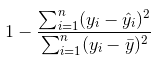When the y-intercept is forced to 0, R-squared is derived using this equation instead: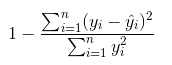In the latter case, the equation will not necessarily match Excel. This is because R-squared is not well defined in this case, and Tableau's behavior matches that of R instead of that of Excel.

Note: The R-Squared value for a linear trend line model is equivalent to the square of the result from the CORR function. See Tableau Functions (Alphabetical)(Link opens in a new window) for syntax and examples for CORR.

#### Standard error

The square root of the MSE of the full model. An estimate of the standard deviation (variability) of the "random errors" in the model formula.

#### p-value (significance)

The probability that an F random variable with the above degrees of freedom exceeds the observed F in this row of the Analysis of Variance table.

#### Analysis of Variance

This table, also known as the ANOVA table, lists information for each factor in the trend line model. The values are a comparison of the model without the factor in question to the entire model, which includes all factors.

#### Individual trend lines

This table provides information about each trend line in the view. Looking at the list you can see which, if any, are the most statistically significant. This table also lists coefficient statistics for each trend line. A row describes each coefficient in each trend line model. For example, a linear model with an intercept requires two rows for each trend line. In the Line column, the p-value and the DF for each line span all the coefficient rows. The DF column under the shows the residual degrees of freedom available during the estimation of each line.

#### Terms

The name of the independent term.

#### Value

The estimated value of the coefficient for the independent term.

#### StdErr

A measure of the spread of the sampling distribution of the coefficient estimate. This error shrinks as the quality and quantity of the information used in the estimate grows.

#### t-value

The statistic used to test the null hypothesis that the true value of the coefficient is zero.

#### p-value

The probability of observing a t-value that large or larger in magnitude if the true value of the coefficient is zero. So, a p-value of .05 gives us 95% confidence that the true value is not zero.

## Assess Trend Line Significance

To see relevant information for any trend line in the view, hover the cursor over it: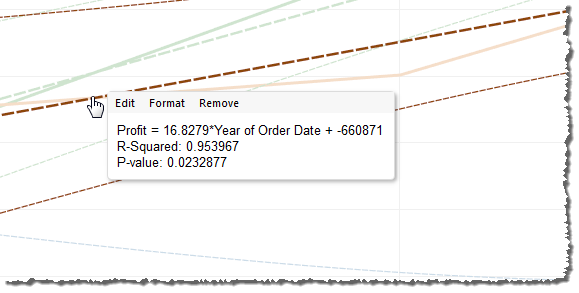The first line in the tooltip shows the equation used to compute a value of Profit from a value of Year of Order Date.

The second line, the R-Squared value, shows the ratio of variance in the data, as explained by the model, to the total variance in the data. For more details, see Trend Line Model Terms.

The third line, the P-value, reports the probability that the equation in the first line was a result of random chance. The smaller the p-value, the more significant the model is. A p-value of 0.05 or less is often considered sufficient.

### Entire Model Significance

Once you’ve added a trend line to a view, you typically want to know the goodness of fit of the model, which is a measure of the quality of the model's predictions. In addition, you may be interested in the significance of each factor contributing to the model. To view these numbers, open the Describe Trend Model dialog box, right-click (Control-click on a Mac) in the view and select Trend Lines >Describe Trend Model.

When you are testing significance, you are concerned with the p-values. The smaller the p-value, the more significant the model or factor is. It is possible to have a model that has statistical significance but which contains an individual trend line or a term of an individual trend line that does not contribute to the overall significance.

Under Trend Lines Model, look for the line that shows the p-value (significance) of the model: The smaller the p-value, the less likely it is that the difference in the unexplained variance between models with and without the relevant measure or measures was a result of random chance.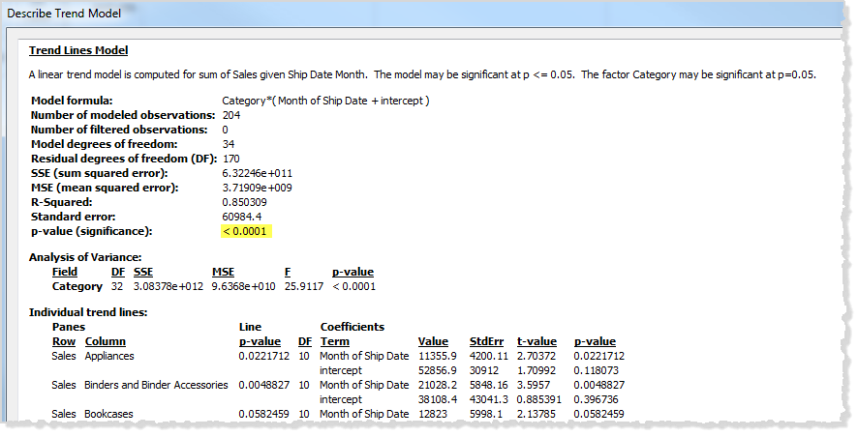This p-value for a model compares the fit of the entire model to the fit of a model composed solely of the grand mean (the average of data in the data view). That is, it assesses the explanatory power of the quantitative term f(x) in the model formula, which can be linear, polynomial, exponential, or logarithmic with the factors fixed. It is common to assess significance using the "95% confidence" rule. Thus, as noted above, a p-value of 0.05 or less is considered good.

### Significance of Categorical Factors

In the Analysis of Variance table, sometimes referred to as the ANOVA table, each field that is used as a factor in the model is listed. For each field, among other values, you can see the p-value. In this case, the p-value indicates how much that field adds to the significance of the entire model. The smaller the p-value the less likely it is that the difference in the unexplained variance between models with and without the field was a result of random chance. The values displayed for each field are derived by comparing the entire model to a model that does not include the field in question.

The following image shows the Analysis of Variance table for a view of quarterly sales for the past two years of three different product categories.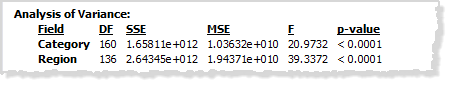As you can see, the p-values for Category and Region are both quite small. Both of these factors are statistically significant in this model.

For information on specific trend line terms, see Trend Line Model Terms.

For ANOVA models, trend lines are defined by the mathematical formula:

``` Y = factor 1 * factor 2 * ...factorN * f(x) + e```

The term `Y` is called the response variable and corresponds to the value you are trying to predict. The term `X` is the explanatory variable, and e (epsilon) is random error. The factors in the expression correspond to the categorical fields in the view. In addition, each factor is represented as a matrix. The `*` is a particular kind of matrix multiplication operator that takes two matrices with the same number of rows and returns a new matrix with the same number of rows. That means that in the expression ```factor 1 * factor 2```, all combinations of the members of factor 1 and factor 2 are introduced. For example, if factor 1 and factor 2 both have three members, then a total of nine variables are introduced into the model formula by this operator.

## Trend Line Assumptions

The p-values reported in Tableau trend lines depend on some assumptions about the data.

The first assumption is that, whenever a test is performed, the model for the mean is (at least approximately) correct.

The second assumption is that the "random errors" referred to in the model formula (see Trend Line Model Types ) are independent across different observations, and that they all have the same distribution. This constraint would be violated if the response variable had much more variability around the true trend line in one category than in another.

### Assumptions Required to Compute Trend Lines

The Assumptions required to compute (using Ordinary Least Squares) each individual trend line are:

• Your model is an accurate functional simplification of the true data generating process (for example, no linear model for a log linear relationship).

• Your errors average to zero and are uncorrelated with your independent variable (for example, no error measuring the independent variable).

• Your errors have constant variance and are not correlated with each other (for example, no increase in error spread as your independent variable increases).

• Explanatory variables are not exact linear functions of each other (perfect multicollinearity).

This section describes some commonly asked questions regarding trend lines in Tableau.

### How do I change the confidence level used in the model?

Tableau does not enforce a confidence level. It simply reports the significance of the whole model, or of a specific field, by showing the p-value. The p-value will measure the probability of obtaining the same trend result without taking the dimensions into account. For example, a trend of sales per time p-value of 0.05 means that there is 5% chance that the same value could be obtained without taking the time into consideration.

### What does it mean if the p-value for the model is significant but the p-value for the specific field in the Analysis of Variance table is not significant?

The p-value in the Analysis of Variance table indicates whether the field adds or detracts from the significance of the entire model. The smaller the p-value the less likely it is that the difference in the unexplained variance between models with and without the field was a result of random chance. The values displayed for each field are derived by comparing the entire model to a model that does not include the field in question. So, for the situation where the p-value for the model is significant but the p-value for the specific field is not, you know that the model is statistically significant, but you cannot be confident that the specific field in question adds anything to it. Consider whether you might not be better off removing the factor from the model.

### What does it mean if the p-value for the specific field in the Analysis of Variance table is significant but the p-value for the model is not significant?

This could happen in a case when there is no "trend" within each pane. For example, the lines are flat, but the mean varies across a given factor.